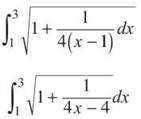﻿ ﻿ARC LENGTH (BC TOPIC ONLY) - Applications of Integration - AP CALCULUS AB & BC REVIEW - Master AP Calculus AB & BC

## Master AP Calculus AB & BC

Part II. AP CALCULUS AB & BC REVIEW

CHAPTER 9. Applications of Integration

ARC LENGTH (BC TOPIC ONLY)

The practice of finding arc length has a long and storied history, stretching all the way back to Noah. Of course, he used cubits, but we can use any unit of measure. It is arguable that Noah had more things on his mind, though: the impending destruction of the human race, ensuring his ark was watertight, and placing layer upon layer of newspaper on the floor. All you have to worry about is passing the AP test, and the good news is that this topic is very easy; all you have to do is memorize a pair of formulas— one for rectangular equations and one for parametric equations.

The Length of a Rectangular Curve (or Arc): If f(x) is an integrable function on [a,b], then the length of the curve from a to b isThat’s all there is to it. Find the derivative and plug it right into the formula—it couldn’t be easier.

Example 8: Find the length of the curve y = sin x from x = 0 to x = 3π/2.

Solution: First, find the derivative (which is very easy).

f(x) = sin x

f'(x) = cos x dx

Now, plug into the formula.NOTE. If the function is in terms of y, the formula can be adjusted. Make sure that a and b are y boundaries, and take the derivative with respect to y instead of x.

This is really not very easy to integrate with our methods, so use the calculator to finish. The arc (or curve) length is approximately 5.730.

It is just as easy to find arc length when you’re dealing with parametric equations; in fact, the formula is very similar to rectangular arc length. The only less obvious difference is that the boundaries of the definite integral in parametric arc length are t-values, not x or y values.

The Length of a Parametric Curve (or Arc): If a and b are t-values for a parametric function, its length between those t-values is given byThe hardest part of differentiating parametric functions is finding dy/dx. Deriving with respect to t is so easy that it’s just silly.

TIP. Many of the integrals you end up with when finding arc length are difficult and have been handed quite a beating with the ugly stick. Don’t expect to be able to solve them all by hand.

Example 9: If a particle moves along the graph defined by x = cos t and y = sin t, how far does the particle move from t = 0 to t = 3π/4? Verify your result geometrically.

Solution: Since the particle moves along the curve, we are just finding the length of the curve. Begin by finding the derivatives.Now, substitute these values into the arc-length formula.According to the Mamma Theorem, the contents of the radical are equal to 1:NOTE. It’s unlikely that you will be asked on the AP text to verify your result geometrically, as this problem does. However, doing so will help you as you learn this topic.

To justify this geometrically, you must realize that the parametric equations result in a circle of radius 1 (the unit circle, actually). The arc length from 0 to 3π/4 represents 3/8 of the circumference of the circle.The circumference of the unit circle is

2πr = 2π ≈ 6.283185307

and 3/8 of that total is 2.35619449; this matches our answer above.

EXERCISE 5

Directions: Solve each of the following problems. Decide which is the best of the choices given and indicate your responses in the book.

YOU MAY USE A GRAPHING CALCULATOR FOR PROBLEMS 2 THROUGH 5.

1. What is the length of the curve y = ln (sin x) from x = π/4 to x = π/2?

2. Find the arc length offrom x = 1 to x = 3.

3. How long is the curve defined by x = y3 — 4 y + 2 from y = —1 to y = 1?

4. Find the length of the parametric curve defined by, y = t2 + t from t = 1 to t = 5.

5. Find the perimeter of the ellipseusing parametric equations.

1. Begin by finding y':The arc length will beThe Pappa Theorem allows you to replace the contents of the radical with csc2x.2. Again, find the derivative first.The arc length will beEvaluate this with your graphing calculator to get 2.562.

3. Even though everything is in terms of y, it would be the exact same problem if every variable were an x.The arc length will be 6.361.

4. First, find the derivatives with respect to t:The arc length will be as follows:NOTE. You could also solve problem 5 using the rectangular formula for arc length.

5. The first order of business is to rewrite the ellipse in parametric form. To do this, remember your Mamma.

cos2t + sin2t = 1

Compare that equation with the ellipse in standard form.You can set cos t = x/2 and sin t = y/3, according to Mamma. Now, solve the new equations for x and y to get the parametric form of the ellipse:

x = 2 cos t, y = 3 sin t

The ellipse is drawn completely from t = 0 to t = 2π, so you can use those values to bound the definite integral. However, exactly half of the integral is drawn from t = 0 to t = π, so let’s evaluate that integral and double it (for a change of pace). Both answers will be the same.The perimeter is 2 ∙ 11.05174608 = 22.103.

﻿